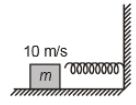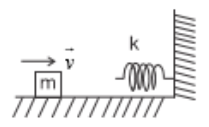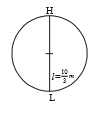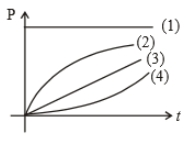A spring 40 mm long is stretched by the application of a force. If 10 N force required to stretch the spring through 1 mm, then work done in stretching the spring through 40 mm is

(a) 84J

(b) 68J

(c) 23J

(d) 8J

Concept Questions :-

Elastic potential energy
NEET 2021 - Achiever Batch - Aryan Raj Singh

Difficulty Level:

Two springs with spring constants ${k}_{1}$ = 1500 N/m and ${k}_{2}$ = 3000 N/m are stretched by the same force. The ratio of potential energy stored in the springs will be

1. 2:1

2. 1:2

3. 4:1

4. 1:4

Concept Questions :-

Elastic potential energy
NEET 2021 - Achiever Batch - Aryan Raj Singh

Difficulty Level:

A block of mass 2 kg moving with velocity of 10 m/s on a smooth surface hits a spring of force constant $80×{10}^{3}$ N/m as shown. The maximum compression in the spring is1. 5 cm

2. 10 cm

3. 15 cm

4. 20 cm

Concept Questions :-

Elastic potential energy
NEET 2021 - Achiever Batch - Aryan Raj Singh

Difficulty Level:

A particle of mass 10 kg is moving with velocity of $10\sqrt{x}$ m/s, where x is displacement . The work done by net force during the displacement of particle from x = 4 to x = 9 m is

1. 1250 J

2. 1000 J

3. 3500 J

4. 2500 J

Concept Questions :-

Concept of work
NEET 2021 - Achiever Batch - Aryan Raj Singh

Difficulty Level:

A body starts moving from rest in straight line under a constant power source. Its displacement in time t is proportional to

(1) ${t}^{1/2}$

(2) t

(3) ${t}^{3/2}$

(4) ${t}^{2}$

Concept Questions :-

Power
NEET 2021 - Achiever Batch - Aryan Raj Singh

Difficulty Level:

A block of mass m = 25 kg on a smooth horizontal surface with a velocity $\stackrel{⇀}{v}$ = 3$m{s}^{-1}$ meets the spring of spring constant k = 100 N/m fixed at one end as shown in the figure. The maximum compression of the spring and velocity of the block as it returns to the original position respectively are1.  1.5m, -3$m{s}^{-1}$

2.  1.5m, 0$m{s}^{-1}$

3.  1.0m, 3$m{s}^{-1}$

4.  0.5m, 2$m{s}^{-1}$

Concept Questions :-

Elastic potential energy
NEET 2021 - Achiever Batch - Aryan Raj Singh

Difficulty Level:

The velocity, given to the block of mass (m), is to rotate it in a circle of radius l. Calculate the height (h) where the block leaves the circle.

(1) $\frac{3l}{2}$

(2) $\frac{4l}{3}$

(3) $\frac{5l}{4}$

(4) None of these

Concept Questions :-

Work-Energy theorem
NEET 2021 - Achiever Batch - Aryan Raj Singh

Difficulty Level:If length of string is

1.  10 m/s

2.  20 m/s

3.  $\frac{10}{\sqrt{2}}\mathrm{m}/\mathrm{s}$

4.

Concept Questions :-

Work-Energy theorem
NEET 2021 - Achiever Batch - Aryan Raj Singh

Difficulty Level:

The relation between velocity (v) and time (t) is $v\propto \sqrt{\mathrm{t}}$, then which one of the following quantity is constant?

1.  Force

2.  Power

3.  Momentum

4.  Kinetic Energy

Concept Questions :-

Power
NEET 2021 - Achiever Batch - Aryan Raj Singh

Difficulty Level:

A particle is moving on the circular path of the radius (R) with centripetal acceleration ${a}_{c}={k}^{2}R{t}^{2}$. Then the correct relation showing power (P) delivered by net force versus time (t) is1. 1

2. 2

3. 3

4. 4

Concept Questions :-

Power